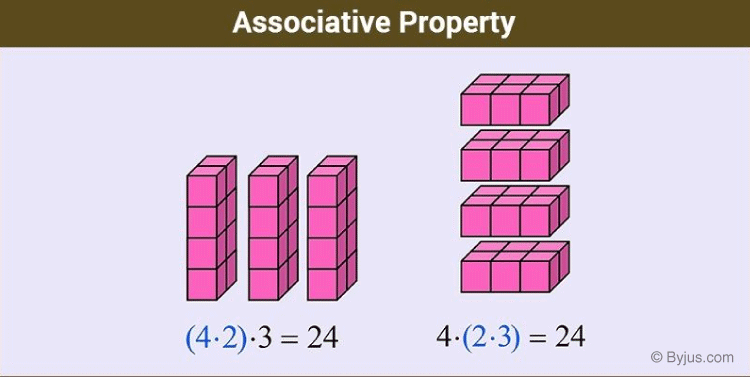Checkout JEE MAINS 2022 Question Paper Analysis : Checkout JEE MAINS 2022 Question Paper Analysis :

# Associative Property

Associative property explains that addition and multiplication of numbers are possible regardless of how they are grouped. By grouping we mean the numbers which are given inside the parenthesis (). Suppose you are adding three numbers, say 2, 5, 6, altogether. Then even if we group the numbers in addition procedures such as 2 + (5 + 6) or (2 + 5) + 6, in both the ways the result will be the same. The same rule applies to multiplication, i.e., 2 x (5 x 6) = (2 x 5) x 6. This property is almost similar to commutative property, where only two numbers are used.

### Associative Property of-

2 + (5 + 6) = (2 + 5) + 6

2 + 11 = 7 + 6

13 = 13

#### Multiplication

2 × (5 × 6) = (2 × 5) × 6

2 × 30 = 10 × 6

60 = 60

Mathematical equations have their own manipulative principles. These principles or properties help us to solve such equations. Basically, there are three properties which outline the backbone of mathematics and these properties are used to perform different arithmetic operations. They are:

• Associative property
• Commutative property
• Distributive property

## Associative Property Definition

Associative as the name implies, means grouping. Origin of the term associative is from the word “associate”. Basic mathematical operations which can be performed using associate property are addition and multiplication. This is normally applicable to more than 2 numbers.

As in case of Commutative property, the order of grouping does not matter in Associative property. It will not alter the result. The grouping of number can be done in parenthesis irrespective of the order of terms. Thus, the associative law expresses that it doesn’t make a difference which part of the operation is carried out first; the answer will be the same.

Note: Both associative and commutative property is applicable for addition and multiplication only.The addition follows associative property i.e. regardless of how numbers are parenthesized the final sum of the numbers will be the same. Associative property of addition states that:

(x+y)+z = x+(y+z)

Let us say, we want to add 5+10+4. It can be seen that the answer is 19. Now, let us group the numbers; put 5 and 10 in the bracket. We get,

⇒ (5+10)+4 = 15+4 = 19        (Remember BODMAS rule)

Now, let’s regroup the terms like 10 and 4 in brackets;

⇒  5+(10+4) = 5 + 14 = 19

Yes, it can be seen that the sum in both cases are the same. This is the associative property of addition.

Let us see some more examples.

(1) 3+(2+1) = (3+2)+1

3+3 = 5+1

6 = 6

L.H.S = R.H.S

(2) 4+(-6+2) = [4 + (-6)] + 2

4 + (-4) = [4-6] + 2

4-4 = -2+2

0 = 0

L.H.S = R.H.S

### Associative Property for Multiplication

Rule for the associative property of multiplication is:

(xy) z = x (yz)

On solving 5×3×2, we get 30 as a product. Now as in addition, let’s group the terms:

⇒ (5 × 3) × 2 = 15 × 2 = 30         (BODMAS rule)

After regrouping,

⇒ 5 × (3 × 2) = 5 × 6 = 30

Products will be the same.

Thus, addition and multiplication are associative in nature but subtraction and division are not associative.

For example, divide 100 ÷ 10 ÷ 5

⇒ (100 ÷ 10) ÷ 5 ≠ 100 ÷ (10 ÷ 5)

⇒ (10) ÷ 5 ≠ 100 ÷ (2)

⇒ 2 ≠ 50

Subtract, 3 − 2 − 1

⇒ (3 − 2) − 1 ≠ 3 − (2 − 1)

⇒ (1) – 1 ≠ 3 − (1)

⇒ 0 ≠ 2

Hence, proved the associative property is not applicable for subtraction and division methods.

## Associative property of Rational Numbers

Suppose a/b, c/d and e/f are rational, then the associativity of addition can be written as:

(a/b) + [(c/d) + (e/f)] = [(a/b) + (c/d)] + (e/f)

Similarly, the associativity of multiplication can be written as:

(a/b) × [(c/d) × (e/f)] = [(a/b) × (c/d)] × (e/f)

Example: Show that (½) + [(¾) + (⅚)] = [(½) + (¾)] + (⅚) and (½) × [(¾) × (⅚)] = [(½) × (¾)] × (⅚).

Solution: (1/2) + [(3/4) + (5/6)] = (1/2) + [(9 + 10)/12]

= (1/2) + (19/12)

= (6 + 19)/12

= 25/12

[(1/2) + (3/4)] + (5/6) = [(2 + 3)/4] + (5/6)

= (5/4) + (5/6)

= (15 + 10)/12

= 25/12

Therefore, (½) + [(¾) + (⅚)] = [(½) + (¾)] + (⅚)

Now, (1/2) × [(3/4) × (5/6)] = (1/2) × (15/24) = 15/48 = 5/16

[(1/2) × (3/4)] × (5/6) = (3/8) × (5/6) = 15/48 = 5/16

Therefore, (½) × [(¾) × (⅚)] = [(½) × (¾)] × (⅚)